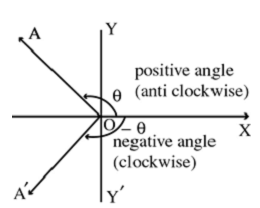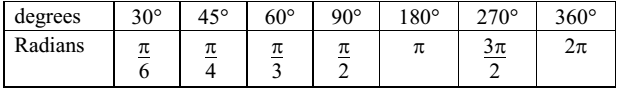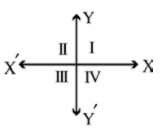### Angle and Measurement

An angle is defined as the amount of rotation of a revolving line from the initial position to the terminal position. Counter-clockwise rotations are called positive and the clockwise are called negative.One complete rotation = 360°. If there is no rotation, the measure of the angle is 0°.

One radian is the measure of an angle subtended at the centre O of a circle of radius r by an arc of length r.Let X′OX and YOY′ be two lines at right angles to each other. X′OX and YOY′ are called as x-axis and y-axis respectively. These axes divide the entire plane into four equal parts, called quadrants.The parts XOY, YOX′, X′OY′and Y′OX are known as the first, the second, the third and the fourth quadrant respectively.

Angle in standard position

If the vertex of an angle is at O and its initial side lies along x-axis, then the angle is said to be in standard position.